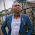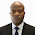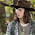## Saturday, February 23, 2019

Shear failure usually occurs in form diagonal cracks, and must be checked under ultimate limit state during the structural design of buildings. In reinforced concrete buildings, shear is resisted using links (stirrups). When a design is failing in shear, the following solutions can be adopted;

(1) Increasing the depth of the beam
(2) Increasing the width of the beam
(3) Increasing the area of shear reinforcement provided and/or reducing the spacing of the links.

It is rare to modify tension reinforcement for the purpose of shear resistance.

In this post, a beam is loaded at ultimate limit state as shown above. We are to design support B for shear according to Eurocode 2 and BS 8110. The preliminary design data is given below.

Design Data:
Concrete Strength = 25 N/mm2
Grade of steel = 500 N/mm2
Width of beam = 300 mm
Depth of beam = 750 mm
Concrete cover = 35 mm

FLEXURAL DESIGN OF SUPPORT B
MEd = 794.5 KN.m

Effective depth (d) = h – Cnom – ϕ/2 - ϕlinks
Assuming ϕ25 mm bars will be employed for the main bars, and ϕ10 mm bars for the stirrups (links)
d = 750 - 35 - (25/2) -10 = 693 mm

k = MEd/(fckbd2) = (794.5 × 106)/(25 × 300 × 6932) = 0.220
Since k > 0.167, compression reinforcement required

Area of compression reinforcement AS2 = (MEd - MRd) / (0.87fyk (d - d2))

MRd = 0.167fckbd2 = (0.167 × 25 × 300 × 6932) × 10-6 = 602 kNm

d2 = 35 + 12.5 + 10 = 57.5 mm

AS2 = ((794.5 – 602) × 106) / (0.87 × 460 × (693 – 57.5)) = 757 mm2
Provide 4H16 Bottom (Asprov = 804 mm2)

Area of tension reinforcement As1 = MRd / (0.87fyk z) + AS2
Where z = d[0.5+ √(0.25 - 0.882k')]
k’ = 0.167
z = d[0.5+ √((0.25 - 0.882(0.167))] = 0.82d

As1 = MRd / (0.87fyk z) + AS2 = ( 602 × 106) / (0.87 × 500 × 0.82 × 693) + 757 mm2 = 3192 mm2

Provide 7H25  TOP (ASprov = 3437 mm2)

SHEAR DESIGN ACCORDING EC2
Support B; VEd = 814 kN

VRd,c = [CRd,c.k.(100ρ1 fck)(1/3) + k1cp]bw.d ≥ (Vmin + k1cp) bw.d

CRd,c = 0.18/γc = 0.18/1.5 = 0.12
k = 1 + √(200/d) = 1 + √(200/693) = 1.53 < 2.0, therefore, k = 1.53
Vmin = 0.035k(3/2) fck0.5
Vmin = 0.035 × (1.53)1.5 × 250.5 = 0.27 N/mm2
ρ1 = As/bd = 3437/(300 × 693) = 0.0165 < 0.02; Therefore take 0.0165

VRd,c = [0.12 × 1.53 (100 × 0.0165 × 25 )(1/3)] × 300 × 693 = 131887 N = 132 kN

Since VRd,c (132 kN) < VEd (814 kN), shear reinforcement is required.
The compression capacity of the compression strut (VRd,max) assuming θ = 21.8° (cot θ = 2.5)

VRd,max = (bw.z.v1.fcd) / (cot⁡θ + tanθ)
V1 = 0.6(1 - fck/250) = 0.6(1 - 25/250) = 0.54
fcd = (αcc fck) / γc = (0.85 × 25) / 1.5 = 14.167 N/mm2
Let z = 0.9d

VRd,max = [(300 × 0.9 × 693 × 0.54 × 14.167) / (2.5 + 0.4)] × 10-3 = 565 kN

Since VRd,c < VRd,max < VEd
We need to modify the strut angle;
θ = 0.5sin-1[(VRd,max /bwd)/0.153fck(1 - fck/250)]
θ = 0.5sin-1[(565000/(300 × 693))/3.4425] = 26°

Since θ < 45°, section is OK for the applied shear stress

Hence Asw / S = VEd / (0.87 Fyk z cot θ) = 814000 / (0.87 × 500 × 0.9 × 693 × 2.05 ) = 1.463

Minimum shear reinforcement;
Asw / S = ρw,min × bw × sinα (α = 90° for vertical links)
ρw,min = (0.08 × √(fck)) / fyk = (0.08 × √25) / 500 = 0.0008
Asw/Smin = 0.0008 × 300 × 1 = 0.24
Maximum spacing of shear links = 0.75d = 0.75 × 693 = 520 mm

Provide H10mm @ 100 mm c/c as shear links (Asw/S = 1.57) Ok!!!!

As a means of comparison, let us consider the shear design of the same support according to BS 8110, assuming the same area of tension reinforcement was provided.

SHEAR DESIGN BY BS 8110-1:1997
Design of support B

Ultimate shear force at centerline of support
V = 814 KN

Using the shear force at the centreline of support;
Shear stress = V/(bd ) = (814 × 103) / (300 × 693) = 3.915 N/mm2

(3.915 N/mm2 ) < 0.8√fcu (4.00 N/mm2 ). Hence, dimensions of the cross-section is adequate for shear.

Concrete resistance shear stress
vc = 0.632 × (100As/bd)1/3 (400/d)1/4

(100As/bd) = (100 × 3437) / (300 × 693) = 1.653 < 3.0 (See Table 3.8 BS 8110-1;1997)

(400/d)1/4 = (400/693)1/4 = 0.87; But for members with shear reinforcement, this value should not be less than 1. Therefore take value as 1.0

vc = 0.632 × (1.653)1/3 × 1.0 = 0.747 N/mm2

Let us check;
(vc + 0.4)1.147 N/mm2 < v(3.915 N/mm2) < 0.8√fck (4.00 N/mm2)

Let us try 2 legs of T10mm bars (Area of steel provided = 157 mm2)

Asv/Sv = (bv (v - vc))/(0.87 × fyv) = [300 × (3.915 - 0.747)] / (0.87 × 500) = 2.184

Maximum spacing = 0.75d = 0.75 × 693 = 520 mm

Try 3 legs of T10mm
Provide 3 legs Y10mm @ 100 mm c/c links as shear reinforcement (Asv/Sv = 2.34)

We can therefore see that disregarding load factor and flexural design requirements, EC2 is more economical than BS 8110 in shear design, and in this case study by about 33%.

1.You said, (bv(v-vc))/0.95fyv. but u used 0.87fyv why?
And please I wud like u to break it down fr me the reason fr 3 legs I am a bit confused.
Thank u very much.

1.Dear Ovie,

The 0.95 and 0.87 was an oversight which has been corrected. I used 0.87Fy because the design was done with Class C reinforcement, with yield strength of 500 Mpa.

On the other hand, you know links are usually bent into rectangular shape with two legs on each side when installed in beams. This time around, we are introducing one more leg in the middle to assist in shear resistance. Thanks.

2.• Thanks for helping us understand this topic. You have written it in a way that makes it very simple to understand. Thank you so much. BIM Consulting
BIM Solutions
BIM Solutions in UK
Shop Drawings Preparation in UK